# AP Statistics Practice Test 5

### Test Information10 questions23 minutes

1. Fifty oranges of one variety were selected at random and weighed. A 95 percent confidence interval computed from the sample weights to estimate the mean weight of oranges of this variety is (7.58, 8.72) ounces. Which of the following statements is true with respect to the confidence level used?

2. In the jury pool available for this week, 30 percent of potential jurors are women. A particular trial requires that, out of a jury of 12, at least three are women. If a jury of 12 is to be selected at random from the pool, what is the probability it meets the requirements of this trial?

3. The mean daily demand for bread at a popular bakery is 2,500 loaves, with a standard deviation of 225 loaves. Every morning the bakery bakes 3,000 loaves. What is the probability that today it will run out of bread? Assume that the mean daily demand for bread at this bakery is normally distributed.

4. A medicine is known to produce side effects in one in five patients taking it. Suppose a doctor prescribes the medicine to four unrelated patients. What is the probability that none of the patients will develop side effects?

5. An automobile service station performs oil changes and tire replacements, as well as other services. Sixty percent of its customers request an oil change, 30 percent request tire replacements, and 10 percent request both. A customer requests an oil change. What is the probability this customer does not request tire replacements?

6. The following boxplot summarizes the prices of books at a bookstore.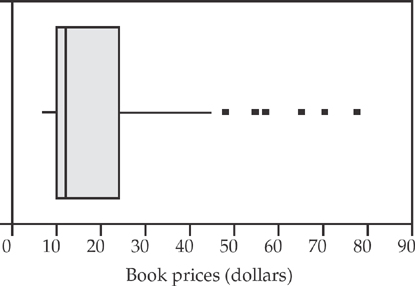Which of the following is true about the prices of books at this store?

7. Random variable X is normally distributed, with a mean of 25 and a standard deviation of 4. Which of the following is the approximate interquartile range for this distribution?

8. An experiment was designed to test the effects of three different types of paint on the durability of wooden toys. Because boys and girls tend to play differently with toys, a randomly selected group of children was divided into two groups by sex. Which of the following statements about this experiment is true?

9. The following graph summarizes data collected on annual rainfall in two cities for the past 150 years.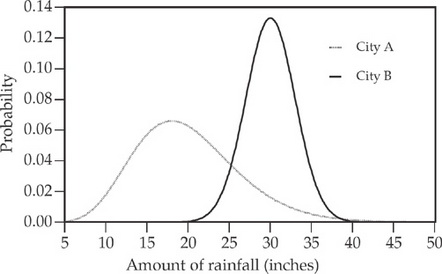Which of the following conclusions can be made from this graph?

10. According to the central limit theorem, regardless of the population distribution, the sample mean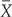is approximately normally distributed with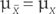and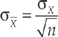,